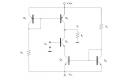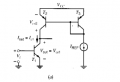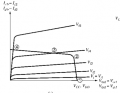# Possible uses for this circuit (common emitter, active load and active bias)

#### diffpair

Joined Dec 19, 2020
1
Hello. I've been studying pic related for some time:At first glance, it's a common emitter amplifier which has both an active load (given by mirrors Q4-Q5) and is biased at an ICQ set by the mirror Q2-Q3. Furthermore, the reference current for each mirror is set by resistors R1, and R2. I understand that for all transistors to work in the linear region, one would need for both reference currents to be equal, that is, assuming everything ideal (forget about building it for now), R1=R2 for it to function as a linear amplifier. If I do the analysis on paper, assuming a small signal vi at Q1, I get that for no load (that is, RL=infinite), the voltage gain is around 0.7, and for RL=13K, around 0.2.

Now, after reading old topics from the forum of similar circuits but biased with a Resistor RE in the emitter of Q1 instead of with a current source, I understand that unless one provides a DC feedback, then this circuit would probably never function, as any mismatch in R1 and R2 would cause one of the transistors to saturate, thus leaving the linear operation region. Then, this circuit would probably not work as is as a linear amplifier. My question, then, is if anybody ever worked with this circuit before and knows what it might be used for (besides as a linear amplifier, which again, and please correct me if I'm wrong, would probably not work due to components mismatches).

I've been reading Gray & Meyer's Analog Circuits, and in the section in which it deals with current mirrors as active loads, I noticed two interesting things: one, in all the examples provided, there's never been a case in which the emitter and collector of a transistor have different current sources, like in this circuit. Also, in most of them, there's only a positive VCC suply, whereas in this circuit there's a dual supply at work (I imagine because one is an npn mirror and the other a pnp, but I'm still curious why this might be). Also, I've been reading that, when an active load is present, the load 'line' changes into a load curve, which makes sense, since the load now is a transistor. Furtheremore, the shape of the output characteristics is the one shown here (this is for a common emitter with active load whose emitter is grounded):Which again, makes sense, since basically the load curve is the output characteristic of the transistor acting as load, mirrored and shifted VCC. Then the Q point is set with the intersection of both curves (The Q points needs to be in the region in which both curves are linear for both transistors to be in the linear operating mode and achieve a high gain, I wonder if there is any use in biasing so that the load transistor is in the saturation region). Interestingly, if I applied this to my circuit (and I might be wrong here) I could essentially select which two curves are intersecting. With R1 I could set the value of ICQ (the collector current of Q1) and, thus, which output curve I'm taking. With R2 I could set the output curve of the load transistor (Q5), and thus select which load curve I'm taking. If this is true, then it's great, but I'll admit I'm not sure how useful it could be. Could this be use for some sort of measurement, for testing something? I think I still lack experience to hazard a guess. Plus, unless I'm mistaken, the output resistance of Q4 is independent of R4, and around VA/IC4, being VA the Early Voltage., so I guess this wouldn't work as a sort of programmable load.

In any case, thanks for reading, and sorry for the long post. As a short recap, I have the circuit in the first picture, and am trying to find out what it could be used for. Using two different current mirrors, one for the biasing and another for the active load, seems to me strange, as I haven't seen anything similar in Gray and Meyer's book (or online for that matter). As such, due to how little likely it would be for this circuit to actually work as an amplifier if built-due to resistances mismatches- I'm thinking its uses lie elsewhere. I think the ability to sort of select which load curve Q1 is seeing by varying R2, and which ICQ Q1 has by varying R1, and thus move around the output characteristics in different Q points based on the intersections of the curves selected, might be a key in understanding what the circuit does. However, this could lead to Q points in which the load transistor is saturated. I'm wondering if anybody ever worked with this or something similar, and might know what it could be used for.

Thanks again.